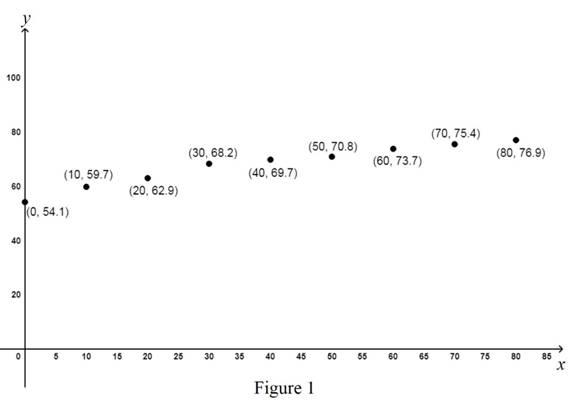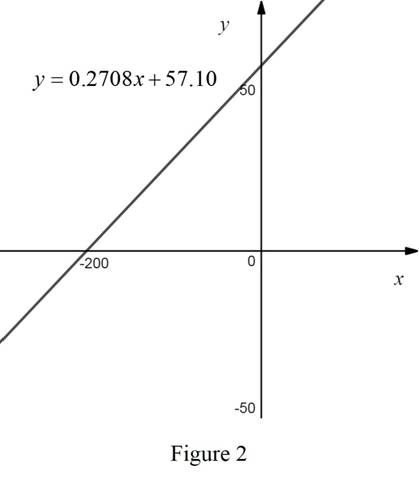# To graph: The scatter plot of the data.### Precalculus: Mathematics for Calcu...

6th Edition
Stewart + 5 others
Publisher: Cengage Learning
ISBN: 9780840068071### Precalculus: Mathematics for Calcu...

6th Edition
Stewart + 5 others
Publisher: Cengage Learning
ISBN: 9780840068071

#### Solutions

Chapter 1, Problem 9P

(a)

To determine

## To graph: The scatter plot of the data.

Expert Solution

### Explanation of Solution

Graph:

Consider the Year as the x coordinates and average life expectancy as the y coordinates.

Let x = Year – 1920.

The scatter plots of the given data are shown below in Figure 1.In Figure 1, all the points are plotted on the graph.

The year 1920 is corresponds to 0 and 2000 is corresponds to 80.

(b)

To determine

### To find: The regression line that represents the given data and draws the graph of the same.

Expert Solution

The regression line that represents the given data is y=0.2708x+57.10 .

### Explanation of Solution

By the use of online calculator, the regression line for the given data obtained is y=0.2708x+57.10 , where average life expectancy is the y-intercept and year is the x-intercept.

Therefore, the regression line that represents the given data is y=0.2708x+57.10 .

The graph that represents the equation y=0.2708x+57.10 is shown below in Figure 1.From Figure 2, the graph for the linear equation y=0.2708x+57.10 is a straight line.

(c)

To determine

### To find: The estimate life expectancy in the year 2006.

Expert Solution

The estimate life expectancy in the year 2006 is 80.4.

### Explanation of Solution

Consider the year 2006.

From part (b), it is obtained that the linear equation that represents the given data is y=0.2708x+57.10 .

Substitute x = 2006 − 1920 in the above equation to compute the life expectancy in the year 2006.

y=0.2708(20061920)+57.10=0.2708(86)+57.10=23.2888+57.10=80.3888

Thus, the estimate life expectancy in the year 2006 is 80.4 years.

(d)

To determine

Expert Solution

### Explanation of Solution

By the use of internet, the actual life expectancy in United states in the year 2006 is 77.69 years.

From part (c), it is obtained that the estimate life expectancy in the year 2006 is 80.4 years.

It is observed that the actual life expectancy is less than the average life expectancy estimated by the use of linear model y=0.2708x+57.10 .

### Have a homework question?

Subscribe to bartleby learn! Ask subject matter experts 30 homework questions each month. Plus, you’ll have access to millions of step-by-step textbook answers!